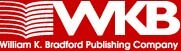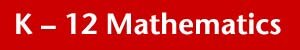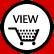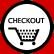Autograph - Graphing Software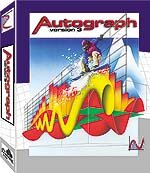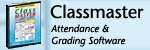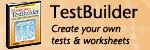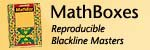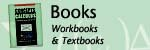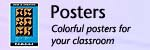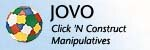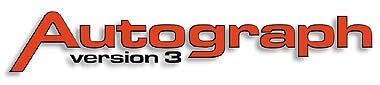Autograph - Graphing Software Developed by: Eastmond Publishing, Ltd. Autograph is the ideal solution for the instructor looking to bring mathematics to life. Whether through true-to-form animations or through student-driven exploration, its powerful features and point-and-click technology will engage all levels of students. There is plenty of evidence now that teaching secondary and college level mathematics with dynamic software can be more effective, can be more efficient, and above all can be more enjoyable for both teacher and student. Autograph leads the way in the use of dependent, selectable mathematical objects to help students come to grips with the basic principles of probability and statistics, and of coordinate geometry in both 2D and 3D. The interactive features allow students to become engaged in the mathematics through teacher-led lessons or in explorations where the answers are found by the students themselves. Example: Students create shapes, and then reflect, slide, and magnify their creations, discovering the relationships of transformational geometry. Autograph spans many levels, including Pre-Algebra, Algebra I, Geometry, Algebra II, Trigonometry, Pre-Calc, Discrete Math, Calculus, Differential Equations, Probability, and Statistics all subjects are covered in one affordable program. Flexibility, ease-of-use, endless possibilities, and value makes Autograph a must for both educators and students.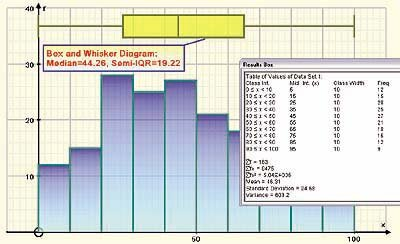Autograph in the Classroom: INTERACTIVE WHITEBOARD Teachers who are fortunate to have this extra layer of interactivity in their classroom are well served by Autograph. Whiteboard Mode Just click on this button and Autograph can be operated effortlessly on screen, and away from the computer. Statistics and Probability... You can enter data directly into Auto-graph, or paste it from Excel or even straight off a web site. Grouped data statistical tables can be generated and exported easily in tabular form to Word. Autograph produces one-dimensional data graphs such as histograms, box-and-whisker diagrams, cumulative frequency diagrams, etc. Or, two-dimensional data graphs such as a scatter diagram. At the 'Advanced' level, an Autograph statistics page allows both probability and statistics objects to be created. There is a range of standard discrete and probability distributions offered as dynamic objects: " Continuous: Rectangular, Normal, User Defined f(x) " Discrete: Rectangular, Binomial, Geometric, Poisson, User Defined All parameters can be varied, with a consequent knock-on effect on any dependent object, e.g., a normal approximation to a binomial, or probability calculations. In the data entry section, samples can be generated from any of the above distributions; a full range of standard statistical diagrams can be created, including line graphs and moving average. In 2D, scatter diagrams can be created from imported data. If a straight line is drawn on the same page, the principle of least-squares regression can be demonstrated. Data for a line graph can be imported in the form (x, f), or as raw data which is then grouped and frequencies calculated automatically.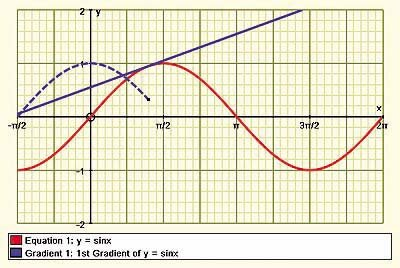2D and 3D Graphing... The possibilities with 2D dynamic objects in coordinate geometry are endless. One of the main top level object types is the equation, which is entered in simple one-line text, with all the necessary symbols at hand. Many equation types can be drawn: straight lines (including the implicit form and inequalities), polynomials and trig functions. Autographs unique Slow Plot option allows plenty of time for a function to be anticipated. Subsequent secondary objects include: intersections, tangents, reflections in y=x, etc. 3D coordinate geometry needs to be seen as a natural extension to 2D, and the study of vectors is the perfect bridge between the two. On a 3D page, Autographs groundbreaking approach offers standard vector notation for lines and planes. Vector cross products are particularly effective! Equations can be in Cartesian form (including implicit) or polar or parametric form; the camera position can be moved freely about the scene.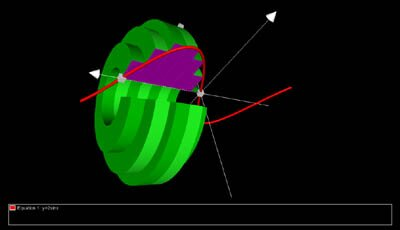ORDER ONLINE: * Unlimited User: Unlimited installs in a single campus location. ** Unlimited User PLUS: Unlimited user plue student personal computer installs. *** Student copy: For schools with a site license of 10 and up. Windows only.